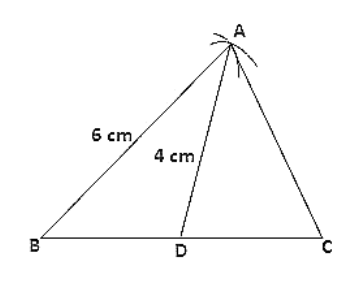# Construct a triangle ABC such that BC = 6 cm,

Question:

Construct a triangle ABC such that BC = 6 cm, AB = 6 cm and median AD = 4 cm.

Solution:

Steps of construction:

1. Draw a line segment BC of 6 cm.

2. Take mid-point O of side BC.

3. With center B and D and radii 6cm and 4cm, draw two arcs which intersect each other at A.

4. Join AB, AD and AC.

Hence △ABC is the required triangle.